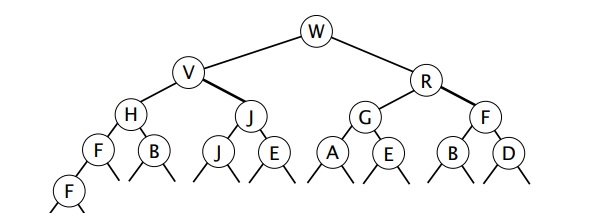### PRIORITY QUEUES STUDY GUIDE

Heaps. A max (min heap is an array representation of a binary tree such that every node is larger (smaller) than all of its children. This definition naturally applies recursively, i.e. a heap of height 5 is composed of two heaps of height 4 plus a parent. Why do we leave the 0th position empty in the array?

Running times of various PQ implementations. Know the running time of the three primary PQ operations for an unordered array, ordered array, and heap implementation.

Heapsort. A max PQ provides an easy algorithm for putting items in ascending order. We simply insert everything into the PQ and then delete the max until the PQ is empty. By using a binary heap, we can sort in place - this is somewhat subtle.

Heapify. Understand how the bottom up heapify process works. Know that it is linear time.

### Recommended Problems

Note: The reason I've given lots of problems here is not because this is a more important topic, but because there are just so many interesting problems.

#### C level

1. Textbook 2.4.2 (assume we'd also like to support delete operations)
2. Textbook 2.4.4
3. Textbook 2.4.11, 2.4.12
4. Textbook 2.4.14
5. Starting from the following max-heap (using the array representation presented in lecture), give the resulting array after each operation:
X 10 7 4 5 6 2 3 0 1
(a) After insert(9)
(b) After delMax(), starting from the original heap (i.e., assuming that (a) has not been performed)

#### B level

1. Continuing from the previous question,
(c) For implementing a max-priority queue, which of the following are advantages of a resizing-array implementation of a heap over a sorted linked list? Circle all that apply.
```    expected time for insert is lower
expected time for delMax is lower
expected storage cost is lower
insert has lower worst-case order of growth
delMax has lower worst-case order of growth
max has lower worst-case order of growth
```
2. Textbook 2.4.7
3. Textbook 2.4.10
4. Textbook 2.4.17, how could you use this technique to find the kth largest item? What would be the runtime of this algorithm in terms of N and k?
5. Textbook 2.4.21
6. Textbook 2.4.27
7. Textbook 2.4.32 (easier than it looks)

8.1. Give the array that represents the correct max heap after deleting the max.
2. Consider the process of creating a max heap from an arbitrary text file containing N integers. What is the order of growth of the run time of the best possible algorithm for this process?